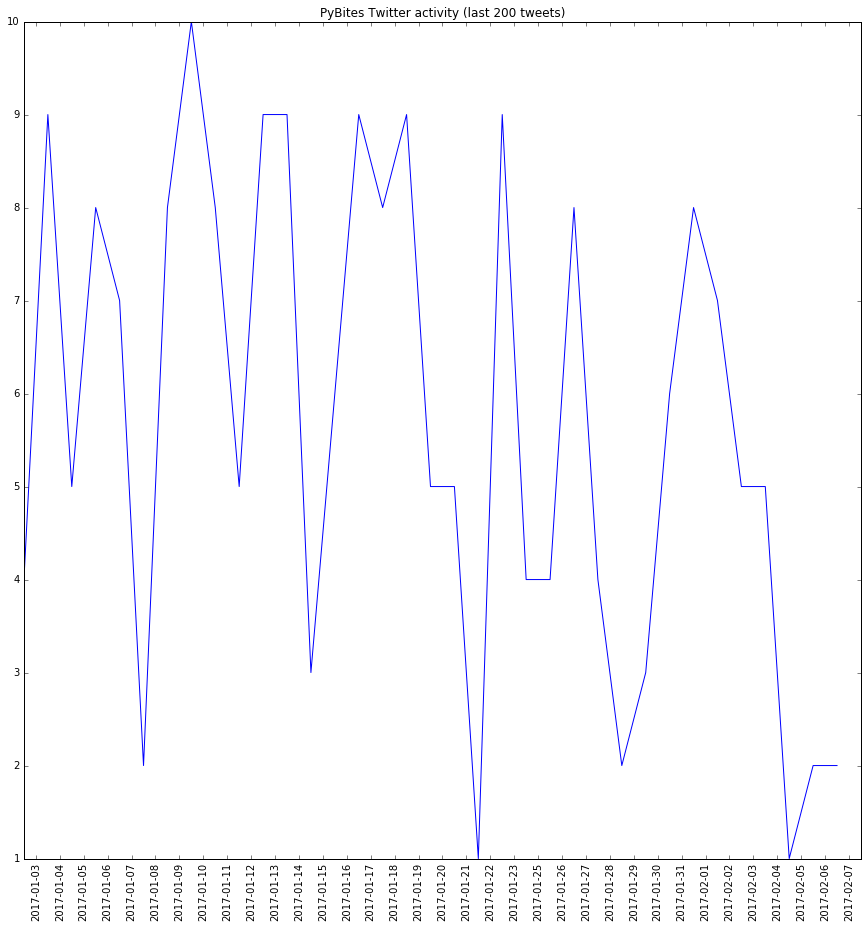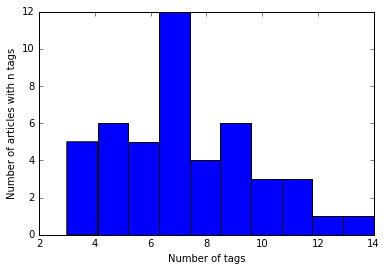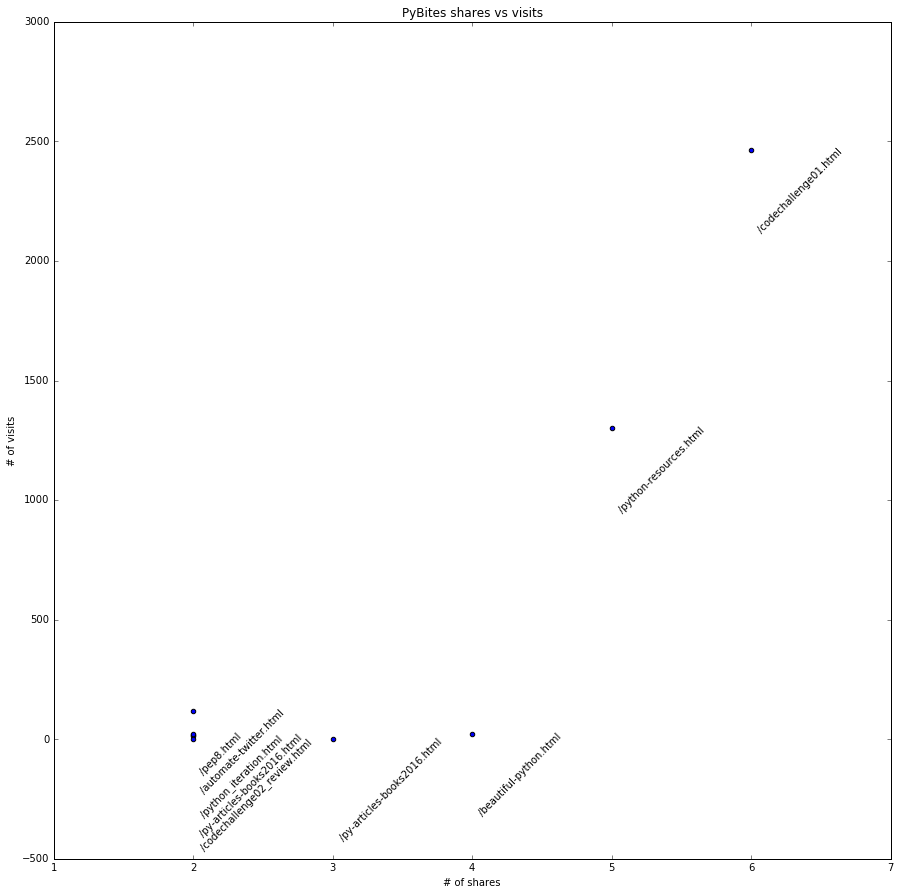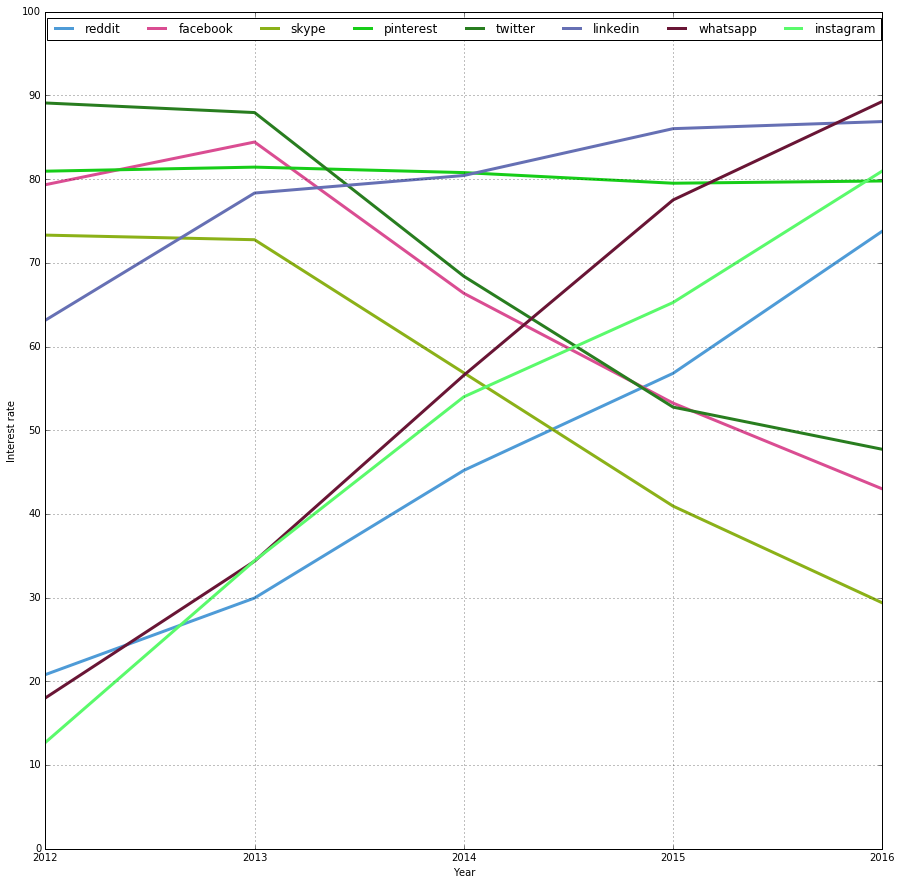# Visualizing website and social media metrics with matplotlib [notebook]

Bob, Wed 08 February 2017, Data

In this notebook I use Matplotlib.pyplot to create simple yet powerful visualizations of PyBites Twitter activity, our blog's tag usage and our website traffic. Lastly I use Google trends to see popularity of various social media.

In :
from collections import Counter, OrderedDict
import csv
import datetime
import itertools
import operator
import random
import re

import feedparser
from matplotlib import pyplot as plt
import numpy as np
import requests

# to show plots inside our Jupyter notebook
%matplotlib inline


### 1. A simple line chart: how often do we tweet per day?¶

For this I ran our challenge 04 code to get a csv with last 200 tweets.

In :
BASE_URL = 'http://projects.bobbelderbos.com/matplotlib/'

def get_csv(url):
with requests.Session() as s:
return list(cr)[1:]

def get_dates(csv):
for row in reversed(csv):
yield row.split()

In :
pybites_csv = get_csv('{}pybites.csv'.format(BASE_URL))
c = Counter(list(get_dates(pybites_csv)))
c_sorted = sorted(c.items(), key=operator.itemgetter(0))
labels, values = zip(*c_sorted)
indexes = np.arange(len(labels))
width = 1

plt.plot(indexes, values)
#plt.bar(indexes, values, width)
plt.xticks(indexes + width * 0.5, labels, rotation=90)

fig = plt.gcf()
fig.set_size_inches(15,15)

plt.title('PyBites Twitter activity (last 200 tweets)')
plt.show() # plt.savefig('file.png') to save to file### 2. Bar chart / distribution of average number of tags PyBites¶

Lets get our feed and make a histogram of the amount of tags used per article.

In :
our_feed = feedparser.parse('http://pybit.es/feeds/all.rss.xml')

In :
tag_count = [len(entry['tags']) for entry in our_feed['entries']]
plt.hist(tag_count)
plt.xlabel("Number of tags")
plt.ylabel("Number of articles with n tags")
plt.show()### 3. Scatterplot of Number of shares vs number of visits PyBites¶

A scatterplot is good for visualizing relationship. See my Brexit analysis for examples, there I related number of leave vs stay votes with demographics (age, education, income, etc.)

In :
top_shares_csv = get_csv('{}TopSharingContent.csv'.format(BASE_URL))
top_visit_csv = get_csv('{}TopVisitContent.csv'.format(BASE_URL))
visits = dict((row, row) for row in top_visit_csv)

In :
# take articles with at least one share
pages, shares = zip(*dict((row, row) for row in top_shares_csv if int(row) > 1).items())
visits = [visits.get(page, 0) for page in pages]

In :
url = re.compile(r'.*pybit.es(/.*?.html).*').sub

plt.scatter(shares, visits)
for page, share, visit in zip(pages, shares, visits):
plt.annotate(url(r'\1', page), xy=(share, visit),
xytext=(5, -5),
textcoords='offset points', rotation=45)
plt.title('PyBites shares vs visits')
plt.xlabel('# of shares')
plt.ylabel('# of visits')

fig = plt.gcf()
fig.set_size_inches(15, 15)

plt.show()### 4. Multi-line plot of social media interest rates (Google Trends)¶

Google Trends lets you measure 'Interest over time':

Numbers represent search interest relative to the highest point on the chart for the given region and time. A value of 100 is the peak popularity for the term. A value of 50 means that the term is half as popular. Likewise a score of 0 means the term was less than 1% as popular as the peak.

Twitter example here. In the next cell I download this for a couple of big social media and aggregate the results, plotting each service.

In :
SOCIAL_MEDIA = 'facebook instagram linkedin pinterest reddit skype twitter whatsapp'.split()
THIS_YEAR = datetime.datetime.now().year

def get_stats(soc_media):
url = '{}{}.csv'.format(BASE_URL, soc_media)
data = dict(get_csv(url)[2:])
for year, days in itertools.groupby(sorted(data), lambda date: date[:4]):
if int(year) < THIS_YEAR:
days = list(days)
yield year, sum(int(data.get(d, 0)) for d in days) / len(days)

soc_med_stats = {}
for sm in SOCIAL_MEDIA:
res = OrderedDict(list(get_stats(sm)))
soc_med_stats[sm] = res

In :
def get_random_color():
"""http://stackoverflow.com/questions/13998901/generating-a-random-hex-color-in-python"""
r = lambda: random.randint(0,255)
yield '#%02X%02X%02X' % (r(),r(),r())

years = list(soc_med_stats[SOCIAL_MEDIA].keys())

plt.figure()
fig = plt.gcf()
fig.set_size_inches(15, 15)
for sm, stats in soc_med_stats.items():
plt.plot(list(stats.keys()), list(stats.values()), label=sm, lw=3, color=next(get_random_color()))
plt.xlim(2012, 2016)
plt.ylim(0, 100)
plt.xticks(range(2012, 2017), ['2012', '2013', '2014', '2015', '2016'])
plt.yticks(range(0,101,10))
plt.xlabel('Year')
plt.ylabel('Interest rate')
plt.legend(loc='upper center', ncol=len(soc_med_stats))
plt.grid(True)
plt.show()Amazing: clearly interest in Instagram, Reddit and Whatsapp grew last couple of years (again this is just one source: Google Trends)

Challenge: make a Programming Language Ranking like Redmonk or PYPL

(no hurries ... we probably will put this as one of the weekly challenges soon ...)

### Training¶

A good video tutorial on this subject is Randy Olson's Data Visualization Basics with Python

### PyBites Python Tips

Do you want to get 250+ concise and applicable Python tips in an ebook that will cost you less than 10 bucks (future updates included), check it out here.

"The discussions are succinct yet thorough enough to give you a solid grasp of the particular problem. I just wish I would have had this book when I started learning Python." - Daniel H

"Bob and Julian are the masters at aggregating these small snippets of code that can really make certain aspects of coding easier." - Jesse B

"This is now my favourite first Python go-to reference." - Anthony L

"Do you ever go on one of those cooking websites for a recipe and have to scroll for what feels like an eternity to get to the ingredients and the 4 steps the recipe actually takes? This is the opposite of that." - Sergio S